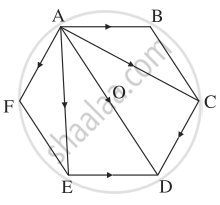# ABCDEF is a regular hexagon. Show that ABACADAEAFAOAB¯+AC¯+AD¯+AE¯+AF¯=6AO¯, where O is the centre of the hexagon. - Mathematics and Statistics

Sum

ABCDEF is a regular hexagon. Show that bar"AB" + bar"AC" + bar"AD" + bar"AE" + bar"AF" = 6bar"AO", where O is the centre of the hexagon.

#### SolutionABCDEF is a regular hexagon.

∴ bar"AB" = bar"ED"  "and"  bar"AF" = bar"CD"

∴ by the triangle law of addition of vectors,

bar"AC" + bar"AF" = bar"AC" + bar"CD" = bar"AD"

bar"AE" + bar"AB" = bar"AE" + bar"ED" = bar"AD"

∴ LHS = bar"AB" +bar"AC" + bar"AD" + bar"AE" + bar"AF"

= bar"AD" + (bar"AC" + bar"AF") + (bar"AE" + bar"AB")

= bar"AD" + bar"AD" + bar"AD"

= 3bar"AD" = 3(2bar"AO")    ....[O is midpoint of AD]

= 6 bar"AO".

Concept: Vectors and Their Types
Is there an error in this question or solution?